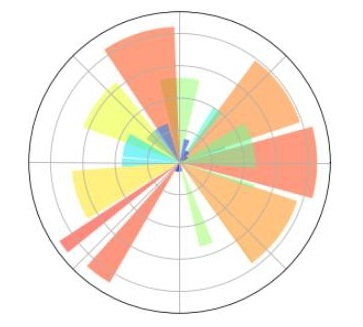# Data visualization with different Charts in Python?

PythonServer Side ProgrammingProgramming

#### Beyond Basic Programming - Intermediate Python

Most Popular

36 Lectures 3 hours

#### Practical Machine Learning using Python

Best Seller

91 Lectures 23.5 hours

#### Practical Data Science using Python

22 Lectures 6 hours

Python provides various easy to use libraries for data visualization. Good thing is that these libraries works with small or large datasets.

Some of the most commonly used python libraries for data visualizations are −

• Matplotlib

• Pandas

• Plotly

• Seaborn

Below we are going to plot different types of visualization chart for one fixed data to better analyse that data.

We are going to analyze below data set to visualize through different charts −

Country or AreaYear(s)VariantValue
India2019Medium
1368737.513
India2019High1378419.072
India2019Low

1359043.965
India2019Constant fertility1373707.838
India2019Instant replacement1366687.871
India2019Zero migration1370868.782
India2019Constant mortality1366282.778
India2019No change

1371221.64
India2019Momentum1367400.614

## Basic Plot

Let's create some basic plots: Line plots, scatter plots and histograms

### Line Plots

Line graphs are plots where a line is drawn to indicate a relationship between a particular set of x and y values.

import matplotlib.pyplot as plt
Year = [2010, 2011, 2012, 2013, 2014, 2015, 2016, 2017, 2018, 2019]
India_Population = [1173108018,1189172906,1205073612,1220800359,1266344631,1309053980,1324171354,1339180127,1354051854,1368737513]
plt.plot(Year, India_Population)
plt.show()

### Output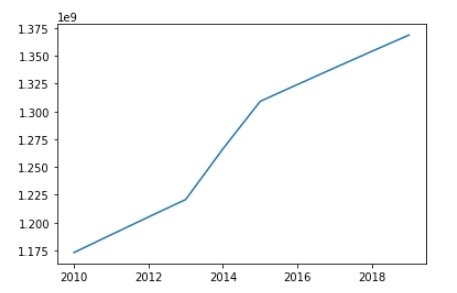### Scatter Plots

Alternatively, you might want to plot quantities with 2 positions as data points.

Consider the same data as for line graph, to create scatter plots we just need to modify one line in the above code −

plt.plot(Year, India_Population,'o')

### Output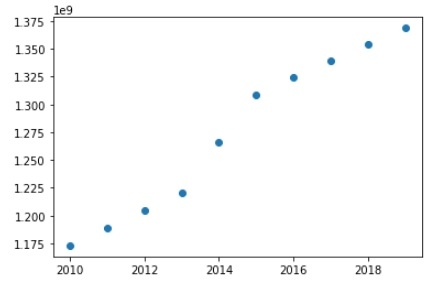### Histogram

Histograms are very often used in science applications and it's highly likely that you will need to plot them at some point. They are very useful to plot distributions.

import pandas as pd
import matplotlib.pyplot as plt
data = [
['India', 2019, 'Medium', 1368737.513],
['India', 2019, 'High', 1378419.072],
['India', 2019, 'Low', 1359043.965],
['India', 2019, 'Constant fertility', 1373707.838],
['India', 2019,'Instant replacement', 1366687.871],
['India', 2019, 'Zero migration', 1370868.782],
['India', 2019,'Constant mortality', 1366282.778],
['India', 2019, 'No change', 1371221.64],
['India', 2019, 'Momentum', 1367400.614],]
df = pd.DataFrame(data, columns = ([ 'Country or Area', 'Year(s)', 'Variant', 'Value']))
df.hist()
plt.show()

### Output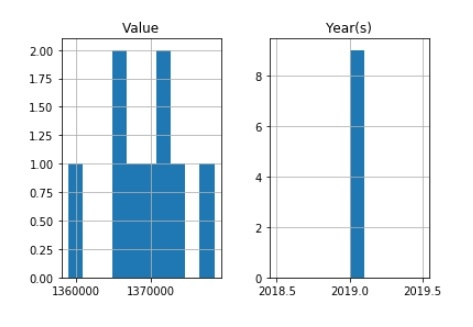### Pie Charts

import numpy as np
import matplotlib.pyplot as plt

n = 25
Z = np.ones(n)
Z[-1] *= 2.5

plt.axes([0.05, 0.05, 0.95, 0.95])
plt.pie(Z, explode = Z*.05, colors = ['%f' % (i/float(n)) for i in range(n)],
wedgeprops = {"linewidth": 1, "edgecolor": "green"})
plt.gca().set_aspect('equal')
plt.xticks([]), plt.yticks([])
plt.show()

### Output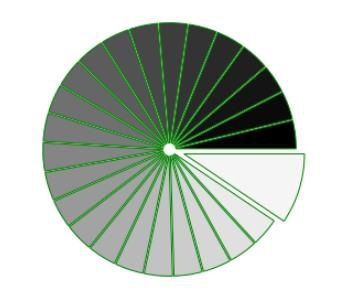### Polar plot

Code:

import numpy as np
import matplotlib.pyplot as plt

ax = plt.axes([0.5,0.05,0.95,0.95], polar=True)

N = 25
theta = np.arange(0.0, 2.5*np.pi, 2.5*np.pi/N)
width = np.pi/4*np.random.rand(N)
bars = plt.bar(theta, radii, width=width, bottom=0.0)

for r,bar in zip(radii, bars):
bar.set_facecolor( plt.cm.jet(r/10.))
bar.set_alpha(0.5)

ax.set_xticklabels([])
ax.set_yticklabels([])

plt.show()

### Output